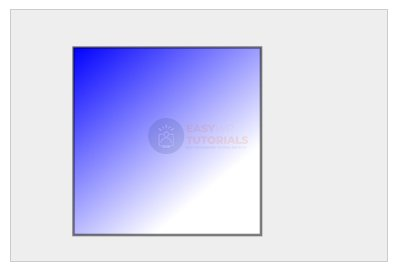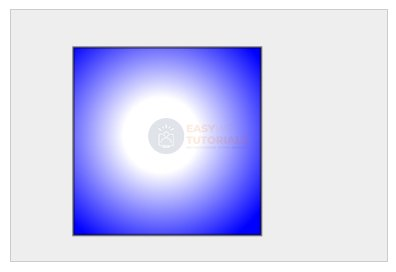# Creating a Gradient in Canvas

A linear gradient is created using the method createLinearGradient(x0, y0, x1, y1), where x0and y0are the start coordinates of the gradient, relative to the upper left corner of the canvas, and x1and y1are the coordinates of the end point of the gradient. For example:

```
```

Also, to create a gradient, you must set the anchor points that determine the color. To do this, the CanvasGradient object uses the addColorStop(offset, color) method , where offset is the offset of the gradient point, and color is its color. For example:

```
```

An offset represents a value between 0 and 1. An offset of 0 represents the beginning of the gradient, and 1 represents the end of the gradient. The color is specified either as a string, as a hexadecimal value, or as an rgb/rgba value.

```<!DOCTYPE html>
<html lang="en">

<meta charset="UTF-8">
<meta http-equiv="X-UA-Compatible" content="IE=edge">
<meta name="viewport" content="width=device-width, initial-scale=1.0">
<title>Canvas in HTML5</title>
<body>
<canvas id="myCanvas" width="300" height="200"
style="background-color:#eee; border:1px solid #ccc;">
Your browser does not support Canvas
</canvas>
</body>
</html>
```In this case, we get a diagonal linear gradient. By manipulating the coordinates, we can achieve a horizontal or vertical gradient.

Matching the x-coordinates of the start and end points creates a horizontal gradient:

```
```

And the coincidence of the y-coordinates of the start and end points creates a horizontal gradient:

```
```

• x0 and y0: coordinates of the center of the first circle
• r0: radius of the first circle
• x1 and y1: coordinates of the center of the second circle
• r1: radius of the second circle

For example:

```
```

And also for the radial gradient, we need to set the anchor color points using the methodaddColorStop()

```<!DOCTYPE html>
<html lang="en">

<meta charset="UTF-8">
<meta http-equiv="X-UA-Compatible" content="IE=edge">
<meta name="viewport" content="width=device-width, initial-scale=1.0">
<title>Canvas in HTML5</title>